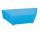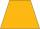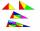Isosceles trapezoid

Calculate the circumference and the contents of the isosceles trapezoid if you know the size of the bases is 8 and 12 cm and the size of the arms is 5 cm.

Result

o =  30 cm
S =  45.826 cm2

Solution:Leave us a comment of example and its solution (i.e. if it is still somewhat unclear...):Be the first to comment!To solve this example are needed these knowledge from mathematics:

Pythagorean theorem is the base for the right triangle calculator. See also our trigonometric triangle calculator.

Next similar examples:

1. Drainage channelThe cross section of the drainage channel is an isosceles trapezoid whose bases have a length of 1.80 m, 0.90 m and arm has length 0.60 meters. Calculate the depth of the channel.
2. EmbankmentPerpendicular cross-section of the embankment around the lake has the shape of an isosceles trapezoid. Calculate the perpendicular cross-section, where bank is 4 m high the upper width is 7 m and the legs are 10 m long.
3. Length ITFind the length (circumference) of an isosceles trapezoid in which the length of the bases a,c and the height h are given: a = 8 cm c = 2 cm h = 4 cm
4. The ditchDitch with cross section of an isosceles trapezoid with bases 2m 6m are deep 1.5m. How long is the slope of the ditch?
5. Parcelparcel has a rectangular shape of a trapezoid with bases 12 m and 10 m and a height 8 m. On parcel was built object with a footprint an isosceles triangle shape with side 4 m and height three-quarters of a meter. What is the area of unbuild parcel?
6. ISO trapezoid v2bases of Isosceles trapezoid measured 12 cm and 5 cm and its perimeter is 21 cm. What is the are of a trapezoid?
7. Trapezoid ABCDCalculate the perimeter of trapezoid ABCD if we know the side c=15, b=19 which is also a height and side d=20.
8. Area of ditchHow great content area will have a section of trapezoidal ditch with a width of 1.6 meters above and below 0.57 meters? The depth of the ditch is 2.08 meters.
9. Right triangle ABCCalculate the perimeter and area of a right triangle ABC, if you know the length of legs 4 cm 5.5 cm and 6.8 cm is hypotenuse.
10. StairwayStairway has 20 steps. Each step has a length of 22 cm and a height of 15 cm. Calculate the length of the handrail of staircases if on the top and bottom exceeds 10 cm.
11. RT 10Area of right triangle is 84 cm2 and one of its cathethus is a=10 cm. Calculate perimeter of the triangle ABC.
12. Four ropesTV transmitter is anchored at a height of 44 meters by four ropes. Each rope is attached at a distance of 55 meters from the heel of the TV transmitter. Calculate how many meters of rope were used in the construction of the transmitter. At each attachment.
13. Broken treeThe tree is broken at 4 meters above the ground and the top of the tree touches the ground at a distance of 5 from the trunk. Calculate the original height of the tree.
14. Chord circleThe circle to the (S, r = 8 cm) are different points A, B connected segment /AB/ = 12 cm. AB mark the middle of S'. Calculate |SS'|. Make the sketch.
15. Center traverseIt is true that the middle traverse bisects the triangle?The double ladder shoulders should be 3 meters long. What height will the upper top of the ladder reach if the lower ends are 1.8 meters apart?A tree at a height of 3 meters broke in the windbreak. Its peak fell 4.5 m from the tree. How tall was the tree?